Lecture 7 - PHY294H

Recommended reading for Lecture 8: Chap. 25, pp661-680

Problem solving - Finding the electric field

We now have two methods for finding the electric field due to either discrete charges or due to continuous distribution of charges. The first is the direct method where we carried out a vector sum of the contributions due to point charges ie. a sum of terms like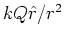. The second method is to use Gauss' law. Here are some guidlines for the best method to solve different types of charge distribution:

Direct method Discrete charge distributions, ring of charge, finite lines of charge, finite sheets of charge.

Gauss' law Spherical shells of charge, sphere's of charge, infinite sheets of charge, infinite rods of charge. Electric field near the surface of a conductor.

Gauss' law calculations

Infinite uniform rod of charge density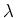Assume the rod has radius a. Use Gauss' law. Choose a cylindrical Gaussian surface, S, of radius r>a and length L. The electric flux is given by,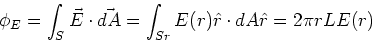(1)

Sr is the curved surface of the cylindrical Gaussian surface. The top and bottom planes of the Gaussian surface do not contribute because the electric field is radial (by symmetry) and is perpendicular to the surface normals at the top and bottom of the cylinder. The enclosed charge is,(2)

Using Gauss' law, ie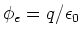, we find,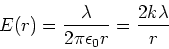(3)

Uniform sphere of charge

Consider a sphere of radius a which has uniform charge densitythroughout. Find the electric field inside and outside of this sphere. Use Gauss' law. Choose the Gaussian surface to be the surface of a sphere. For a surface of radius r, the electric flux is given by,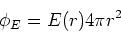(4)

The charge enclosed by this surface changes with r. For r<a, the enclosed charge is given by,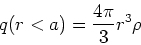(5)

while for r>a we have,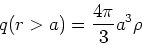(6)

Using Gauss's law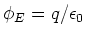, we thus have,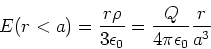(7)

where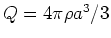is the total charge on the sphere. The electric field outside the sphere of charge is like that of a point charge (shell theorem),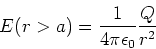(8)

In both cases, the direction of the electric field is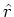.

We also went through problem 24.26

Electric potential energy and electric potential

The electric potential energy (U) is the potential energy due to the electrostatic force. As always we use only differences in potential energy. However we define a reference potential energy and make calculate all differences in potential energy with respect to this reference. In electrostatics, the potential energy is defined to be zero when the charges are an infinite distance apart.

The difference in potential energy in moving a charge between two positions a and b is defined in terms of the work done in moving the charge between these two positions, so that,(9)

where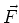is the Coulomb force on the charge and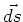is a small element of distance along the path we choose to take from a to b. Note that Eq. (9) does not depend on the path we take. All paths from a to b give the same change in potential energy. Note also that Eq. (9) is true only for conservative forces, ie. systems in which there is no dissipation (e.g. friction).

Instead of using the Coulomb force, we defined the electric field which is the force per unit charge. In the same way we define the electric potential, V, to be the potential energy per unit charge, ie. U=qV. The electric potential is so important it is given its own unit, the volt (V). In terms of the electric potential, equation (9) is,(10)

From this equation we see that the units of voltage are N m/C. We have defined the volt to be the unit of voltage, so we have the relation V = Nm/C or V/m = N/C. So far we have used N/C as the units of electric field. This is usual in electrostatics. However in circuit theory it is usual to use volts/meter (V/m).